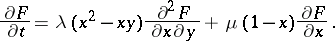# Epidemic process

(diff) ← Older revision | Latest revision (diff) | Newer revision → (diff)

A random process (cf. Stochastic process) that serves as a mathematical model of the spread of some epidemy. One of the simplest such models can be described as a continuous-time Markov process whose states at the momentare the numberof sick persons and the numberof exposed persons. If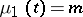and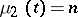, then at the time,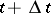,, the transition probability is determined as follows:with probability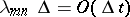;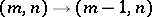with probability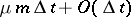. In this case the generating function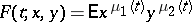satisfies the differential equation